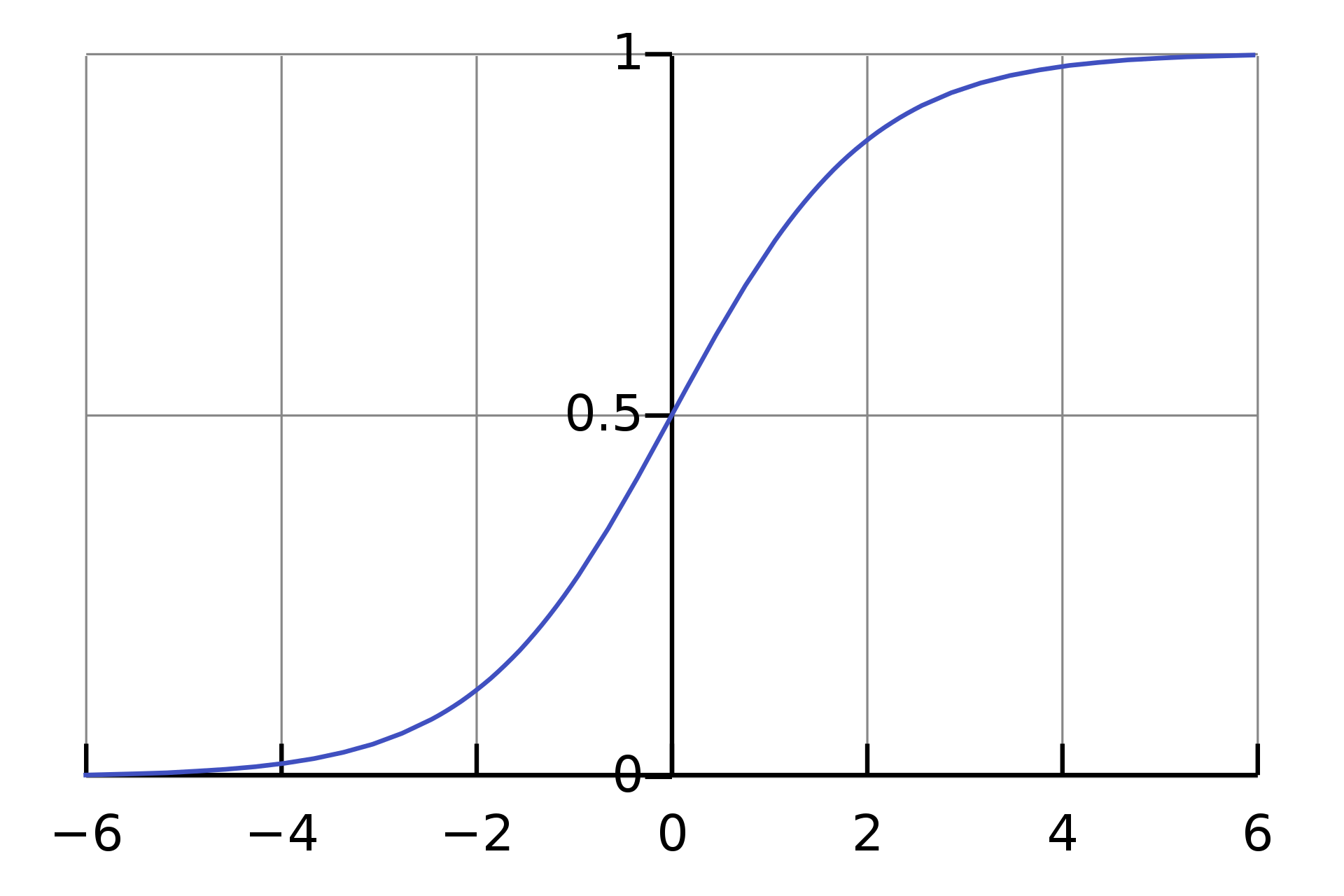# Logistic Regression：互联网领域应用最广的模型

Logistic Regression曾经在互联网业务中被广泛用来进行搜索、推荐和广告的点击预估，可以说是使用频次最多的机器学习模型，也是深度神经网络的基础。在一些机器学习新人面试中，面试官经常会考察Logistic Regression的基本公式、损失函数的推导等问题。

## 从回归到分类• 函数定义域为$(-\infty, +\infty)$，值域为$(0, 1)$
• $z$趋近于$-\infty$时，$g(z)$趋近于$0$；当$z$趋近于$+\infty$时，$g(z)$趋近于$1$；当$z$$0$时，$g(z)$等于$0.5$
• 整个函数呈S形。
• 函数单调可微。

Logistic函数的这些性质决定了它可以将$(-\infty, +\infty)$映射到$(0, 1)$上，加上它在中心点处取值为$0.5$，可以用来进行分类。因为Logistic函数有明确的分界线，$z$小于0的部分将被分为负例（0），$z$大于0的部分将被分为正例（1）。

## Logistic函数二元概率解释

Logistic函数适合表示二分类概率。假设我们将$y$表示为分类时作为正例的可能性，那么$1-y$就是分成负例的可能性。恰好Logistic Regression有如下性质： $\ln \frac{y}{1-y}=w^\top x$

Logistic函数将$(-\infty, +\infty)$映射到了$(0, 1)$上，无论多大或者多小的值，都可以和一个$(0, 1)$区间的概率联系起来，这样就得到了一个概率分布。

## Logistic Regression的最大似然估计

Logistic函数可以和概率联系起来，于是我们可以将$y$视为分类到正例的概率估计：$P(y=1|\boldsymbol{x})$，分类到负例的概率为：$P(y=0|\boldsymbol{x})$$P(y=1|\boldsymbol{x}) = f(\boldsymbol{x})$

$P(y=0|\boldsymbol{x})=1-f(\boldsymbol{x})$

$P(Y=y | \boldsymbol{x};\boldsymbol{w}) =(f (\boldsymbol{x}))^y(1- f (\boldsymbol{x}))^{1-y}$ 由于$y$只有两种可能，即0（负例）和1（正例）：那么如果$y=1$$(1- f (\boldsymbol{x}))^0=1$$P(Y=y | \boldsymbol{x})=(f(\boldsymbol{x}))^1$，如果$y=0$$(f (\boldsymbol{x}))^0=1$$P(Y=y | \boldsymbol{x})=(1-f(\boldsymbol{x}))^1$。上式中，分号和$\boldsymbol{w}$表示，$\boldsymbol{w}$是参数，并不是随机变量。

\begin{aligned} \ell(\boldsymbol{w}) &=\log L(\boldsymbol{w}) \\ &= \sum^m_{i=1} y^{(i)} \log f(\boldsymbol{x}^{(i)})+(1-y^{(i)})\log (1-f(\boldsymbol{x}^{(i)})) \end{aligned} 如何求得上面公式的解？和线性回归一样，我们可以利用梯度上升法。当前目标是最大化似然函数，因此我们要使用梯度上升，不断迭代寻找最大值。具体而言，参数按照下面的方式来更新： $\boldsymbol{w} := \boldsymbol{w} +\alpha \nabla _\boldsymbol{w} \ell(\boldsymbol{w})$ 参数估计中最关键的是得到导数公式。求导之前，我们再回顾一下Logistic Regression： $f(\boldsymbol{x}) = g(\boldsymbol{w}^\top \boldsymbol{x})$ $g(z)= \frac 1 {1+e^{-z}}$

## 分类阈值

Logistic Regression最终返回的是概率，我们可以直接使用这个预测出来的概率，也可以设定阈值，将概率转化成二元分类问题。

## 类别训练数据不均衡问题

• 在垃圾邮件识别场景下，比起正常邮件，垃圾邮件的数目相对较少。
• 在信用卡欺诈检测的场景下，绝大多数交易是正常的，只有少量交易有问题。
• 在机器故障报警场景下，机器绝大多数情况下正常运行，发生故障的时间远小于正常运行的时间。

• 对训练集进行欠采样（Undersampling）或过采样（Oversampling），减少或增加数据，使得训练集正负例数目接近，然后再进行学习。
• 直接使用原始训练集进行学习，但是不再使用0.5作为分类阈值，而是根据业务场景、样本正负例数目对阈值进行一定调整。

1. Andrew Ng：CS229 Lecture Notes
2. 周志华: 机器学习